## What is Harmonic Progression in Mathematics?

### Harmonic Progression (H.P.)

Definition:
A progression is called a harmonic progression (H.P.) if the reciprocals of its terms are in A.P.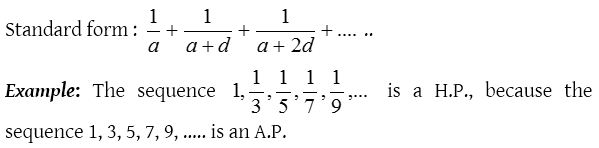### General term of an H.P.### Harmonic mean

If three or more numbers are in H.P., then the numbers lying between the first and last are called harmonic means (H.M.’s) between them. For example 1, 1/3, 1/5, 1/7, 1/9 are in H.P. So 1/3, 1/5 and 1/7 are three H.M.’s between 1 and 1/9.
Also, if a, H, b are in H.P., then H is called harmonic mean between a and b.
(1) Insertion of harmonic means
(i) Single H.M. between a and b $$=\frac { 2ab }{ a+b }$$.
(ii) H, H.M. of n non-zero numbers a1, a2, a3, ….. an is given by(iii) Let a, b be two given numbers. If n numbers H1, H2, H3, ….. Hn are inserted between a and b such that the sequence  a, H1, H2, H3, ….. Hn, b is a H.P., then H1, H2, H3, ….. Hn are called n harmonic means between a and b.Thus, if n harmonic means are inserted between two given numbers a and b, then the common difference of the corresponding A.P. is given by### Properties of H.P.

1. No term of H.P. can be zero.
2. If H is the H.M. between a and b, then### Harmonic Progression Problems with Solutions

1.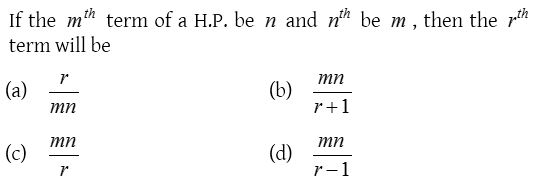Solution: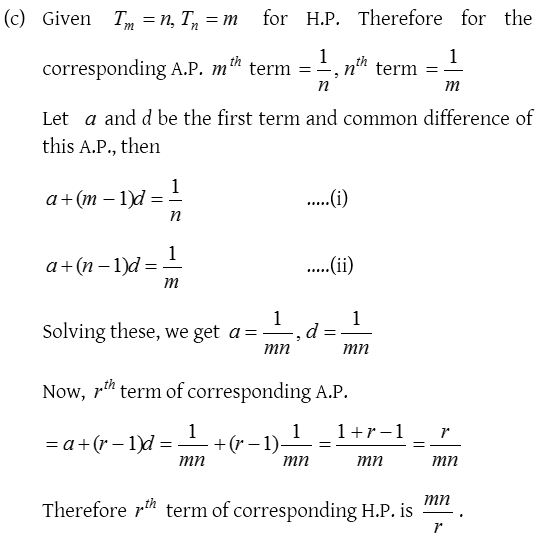2.Solution:3.Solution: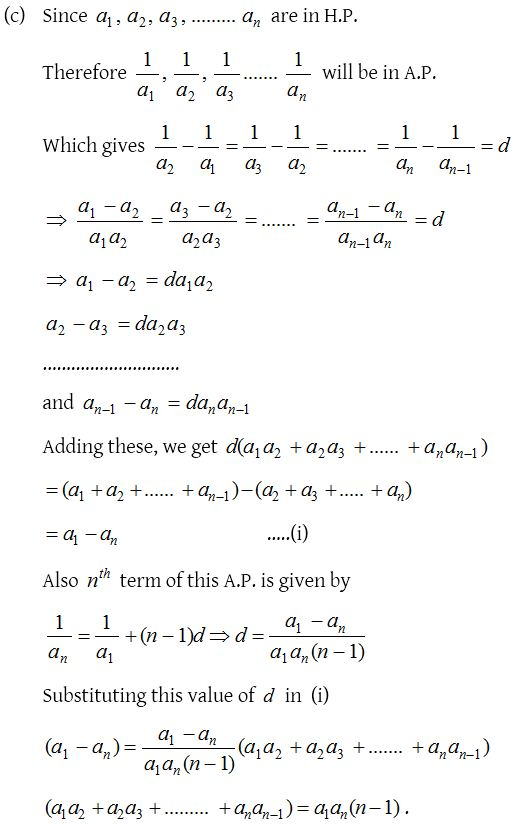4.Solution: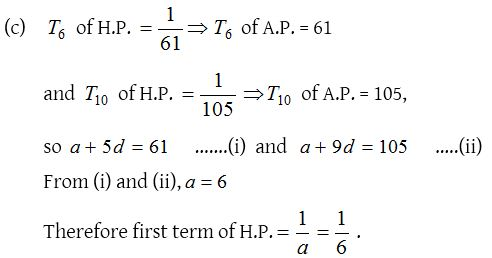5.Solution:6.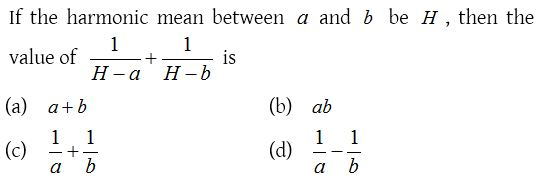Solution:7.Solution: